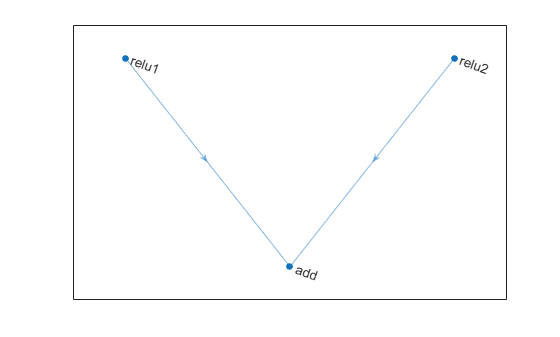# connectLayers

Connect layers in layer graph or network

## Syntax

``lgraphUpdated = connectLayers(lgraph,s,d)``
``netUpdated = connectLayers(net,s,d)``

## Description

example

````lgraphUpdated = connectLayers(lgraph,s,d)` connects the source layer `s` to the destination layer `d` in the layer graph `lgraph`. The updated layer graph, `lgraphUpdated`, contains the same layers as `lgraph` and includes the new connection.```
````netUpdated = connectLayers(net,s,d)` connects the source layer `s` to the destination layer `d` in the `dlnetwork` object `net`. The updated network, `netUpdated`, contains the same layers as `net` and includes the new connection.```

## Examples

collapse all

Create an addition layer with two inputs and the name `'add_1'`.

`add = additionLayer(2,'Name','add_1')`
```add = AdditionLayer with properties: Name: 'add_1' NumInputs: 2 InputNames: {'in1' 'in2'} ```

Create two ReLU layers and connect them to the addition layer. The addition layer sums the outputs from the ReLU layers.

```relu_1 = reluLayer('Name','relu_1'); relu_2 = reluLayer('Name','relu_2'); lgraph = layerGraph; lgraph = addLayers(lgraph,relu_1); lgraph = addLayers(lgraph,relu_2); lgraph = addLayers(lgraph,add); lgraph = connectLayers(lgraph,'relu_1','add_1/in1'); lgraph = connectLayers(lgraph,'relu_2','add_1/in2'); plot(lgraph)```Create a simple directed acyclic graph (DAG) network for deep learning. Train the network to classify images of digits. The simple network in this example consists of:

• A main branch with layers connected sequentially.

• A shortcut connection containing a single 1-by-1 convolutional layer. Shortcut connections enable the parameter gradients to flow more easily from the output layer to the earlier layers of the network.

Create the main branch of the network as a layer array. The addition layer sums multiple inputs element-wise. Specify the number of inputs for the addition layer to sum. To easily add connections later, specify names for the first ReLU layer and the addition layer.

```layers = [ imageInputLayer([28 28 1]) convolution2dLayer(5,16,'Padding','same') batchNormalizationLayer reluLayer('Name','relu_1') convolution2dLayer(3,32,'Padding','same','Stride',2) batchNormalizationLayer reluLayer convolution2dLayer(3,32,'Padding','same') batchNormalizationLayer reluLayer additionLayer(2,'Name','add') averagePooling2dLayer(2,'Stride',2) fullyConnectedLayer(10) softmaxLayer classificationLayer];```

Create a layer graph from the layer array. `layerGraph` connects all the layers in `layers` sequentially. Plot the layer graph.

```lgraph = layerGraph(layers); figure plot(lgraph)```Create the 1-by-1 convolutional layer and add it to the layer graph. Specify the number of convolutional filters and the stride so that the activation size matches the activation size of the third ReLU layer. This arrangement enables the addition layer to add the outputs of the third ReLU layer and the 1-by-1 convolutional layer. To check that the layer is in the graph, plot the layer graph.

```skipConv = convolution2dLayer(1,32,'Stride',2,'Name','skipConv'); lgraph = addLayers(lgraph,skipConv); figure plot(lgraph)```Create the shortcut connection from the `'relu_1'` layer to the `'add'` layer. Because you specified two as the number of inputs to the addition layer when you created it, the layer has two inputs named `'in1'` and `'in2'`. The third ReLU layer is already connected to the `'in1'` input. Connect the `'relu_1'` layer to the `'skipConv'` layer and the `'skipConv'` layer to the `'in2'` input of the `'add'` layer. The addition layer now sums the outputs of the third ReLU layer and the `'skipConv'` layer. To check that the layers are connected correctly, plot the layer graph.

```lgraph = connectLayers(lgraph,'relu_1','skipConv'); lgraph = connectLayers(lgraph,'skipConv','add/in2'); figure plot(lgraph);```Load the training and validation data, which consists of 28-by-28 grayscale images of digits.

```[XTrain,YTrain] = digitTrain4DArrayData; [XValidation,YValidation] = digitTest4DArrayData;```

Specify training options and train the network. `trainNetwork` validates the network using the validation data every `ValidationFrequency` iterations.

```options = trainingOptions('sgdm', ... 'MaxEpochs',8, ... 'Shuffle','every-epoch', ... 'ValidationData',{XValidation,YValidation}, ... 'ValidationFrequency',30, ... 'Verbose',false, ... 'Plots','training-progress'); net = trainNetwork(XTrain,YTrain,lgraph,options);```Display the properties of the trained network. The network is a `DAGNetwork` object.

`net`
```net = DAGNetwork with properties: Layers: [16x1 nnet.cnn.layer.Layer] Connections: [16x2 table] InputNames: {'imageinput'} OutputNames: {'classoutput'} ```

Classify the validation images and calculate the accuracy. The network is very accurate.

```YPredicted = classify(net,XValidation); accuracy = mean(YPredicted == YValidation)```
```accuracy = 0.9934 ```

## Input Arguments

collapse all

Layer graph, specified as a `LayerGraph` object. To create a layer graph, use `layerGraph`.

Neural network, specified as a `dlnetwork` object.

Connection source, specified as a character vector or a string scalar.

• If the source layer has a single output, then `s` is the name of the layer.

• If the source layer has multiple outputs, then `s` is the layer name followed by the character / and the name of the layer output: `'layerName/outputName'`.

Example: `'conv1'`

Example: `'mpool/indices'`

Connection destination, specified as a character vector or a string scalar.

• If the destination layer has a single input, then `d` is the name of the layer.

• If the destination layer has multiple inputs, then `d` is the layer name followed by the character / and the name of the layer input: `'layerName/inputName'`.

Example: `'fc'`

Example: `'addlayer1/in2'`

## Output Arguments

collapse all

Updated layer graph, returned as a `LayerGraph` object.

Updated network, returned as an uninitialized `dlnetwork` object.

To initialize the learnable parameters of a `dlnetwork` object, use the `initialize` function.

The `connectLayers` function does not preserve quantization information. If the input network is a quantized network, then the output network does not contain quantization information.

## Version History

Introduced in R2017b